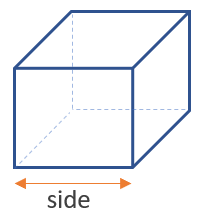# Volume of a Cube Calculator

Use this cube volume calculator to easily calculate the volume of a cube, given its side in any metric: mm, cm, meters, km, inches, feet, yards, miles...

### Calculation results

27 cm3 Cube volume
Share calculator:

Embed this tool:
get code

## Volume of a cube formula

The volume of a cube can be calculated if you know its side length. The formula is then volumecube = side3. This is simply the length of the side multiplied by itself two times. Illustration below:Measuring the side of the cube is easy. The result from the calculation, using our volume of a cube calculator or otherwise, will always be in the length unit used, cubed. So, if you measured it in inches, the result will be in cubic inches. If the length was in feet, the result will be in cubic feet, and so on for cubic yards, cubic miles, cubic millimeters, cubic centimeters, cubic meters. If you need to find the volume in a specific unit, then a unit conversion would be needed which is most easily done to the length of the side, before replacing it in the formula.

## How to calculate the volume of a cube?

This is one of the simplest bodies one can calculate the volume of. You only need a single measurement, then you multiply it by itself and by itself again, equivalent to raising it to the power of 3. Finally, adjust the unit to its cubic equivalent. Simple as that.

In practical situations, you rarely know in advance that something is a cube, so you might still need to measure at least three sides before you can confirm this. If you have a plan or engineering schematic in which the measurements are all given, your task is significantly easier.

## Example: find the volume of a cube

The only variable one needs to know to compute the volume of any cube is the length of one of its sides. Since all sides are equal, it does not matter which side is given exactly. For example, if the length of a side is 5 inches, using the volume equation results in 53 = 5 x 5 x 5 = 125 in3 (cubic inches).

#### Cite this calculator & page

If you'd like to cite this online calculator resource and information as provided on the page, you can use the following citation:
Georgiev G.Z., "Volume of a Cube Calculator", [online] Available at: https://www.gigacalculator.com/calculators/volume-of-cube-calculator.php URL [Accessed Date: 01 Apr, 2023].